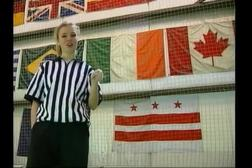PLAY PREVIEW

### Segments in this Video

#### Two Dimensional Kinematics: Introduction(00:36)

FREE PREVIEW

Two dimensional kinematics deals with motion along the x-y plane. This program will cover projectile motion, uniform circular motion, accelerated circular motion and rotational motion.

#### Projectile Motion(15:27)

Review the one dimensional kinematic equation and learn how to find x and y component magnitude. Learn the acceleration due to gravity number and the first through fourth projectile motion equations. View sample problems determining x and y values.

#### Projectile Motion Review(01:30)

An object tossed into the air follows a curved path. Review how to use the first through fourth projectile motion equations to solve a problem.

#### Uniform Circular Motion(04:54)

Learn about centripetal force and centripetal acceleration. View sample problems determining the radius of a circular path and the period of time it takes to complete one orbit.

#### Uniform Circular Motion Review(00:46)

An object moving around a circle is in uniform circular motion with the radius at a constant velocity. Review centripetal acceleration, period, and velocity concepts.

#### Credits: Cinemática Fisica(01:38)

Credits: Cinemática Fisica

For additional digital leasing and purchase options contact a media consultant at 800-257-5126
(press option 3) or sales@films.com.

# Cinemática Fisica

Part of the Series : Standard Deviants Español Fisíca Series
 3-Year Streaming Price: \$129.95

Share

### Description

The Standard Deviants discuss two-dimensional kinematics and motion in a plane, with a look at the role the Big G—gravity—plays in projectile motion. Then we'll show you some handy equations for figuring out projectile motion and uniform circular motion. Kinematics in two dimensions is motion in a plane, the most useful study of motion at the Newtonian level. Projectile motion equations are introduced as well as uniform circular motion and centripetal force and acceleration. Projectile motion covers clowns from cannons as well as rockets, squirt guns, and skiers flying off jumps taking into account the effect of gravity.

Length: 28 minutes

Item#: BVL155187Closed Captioned